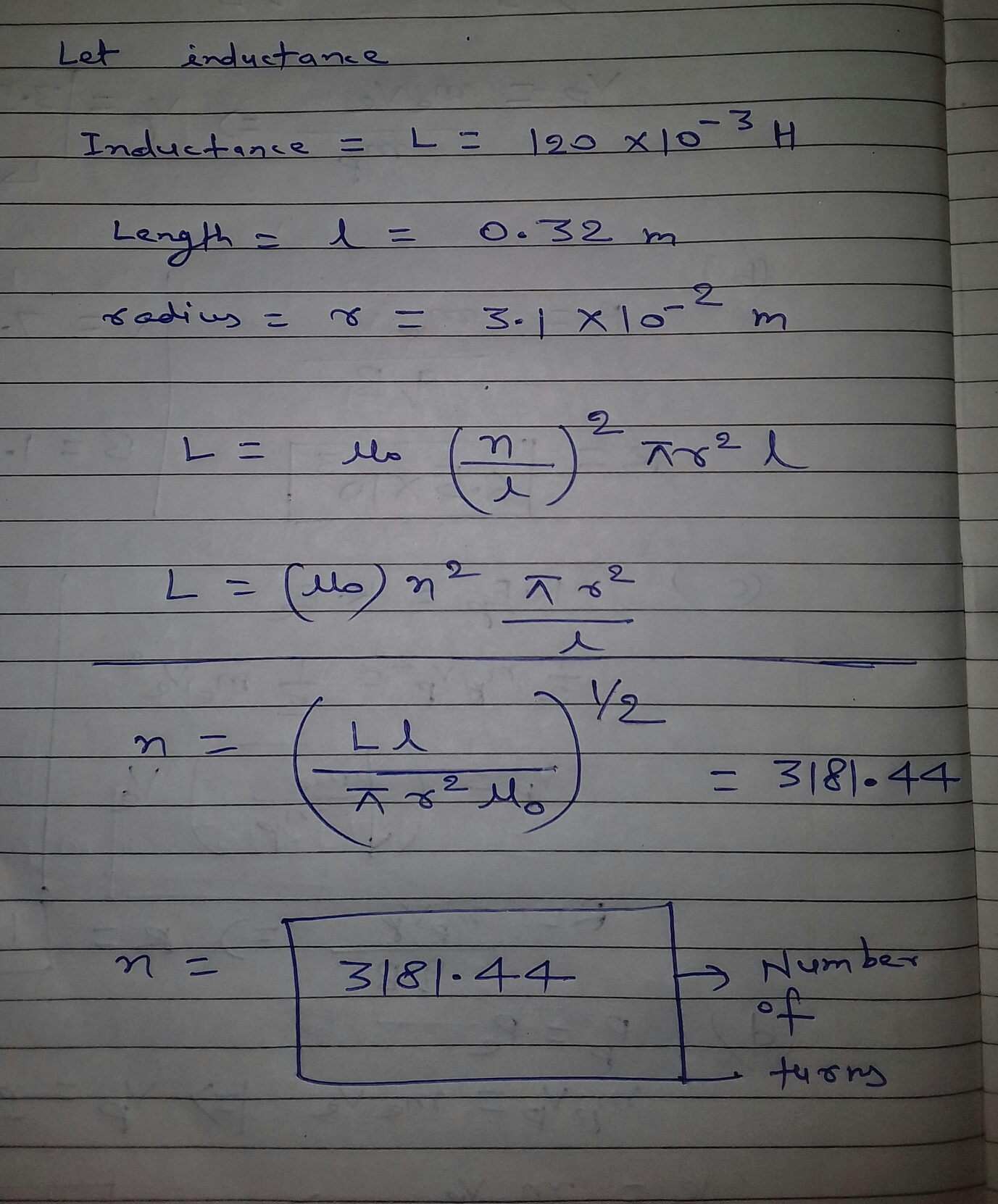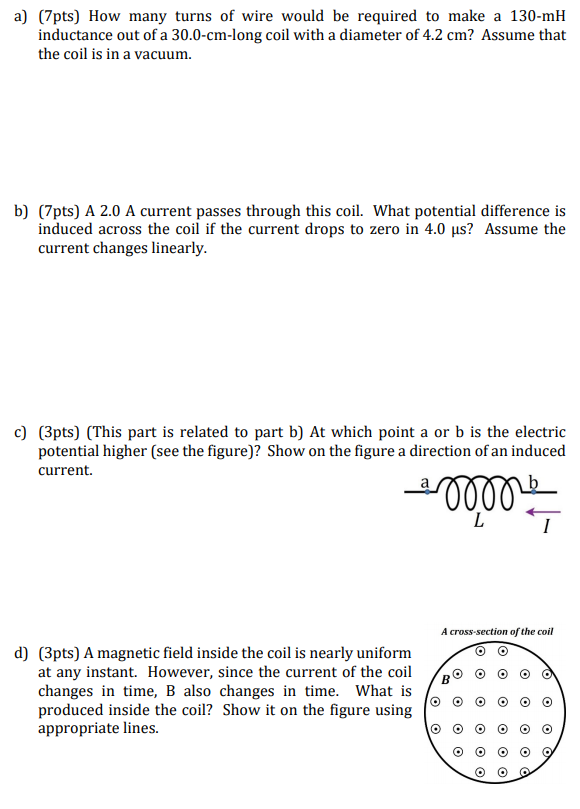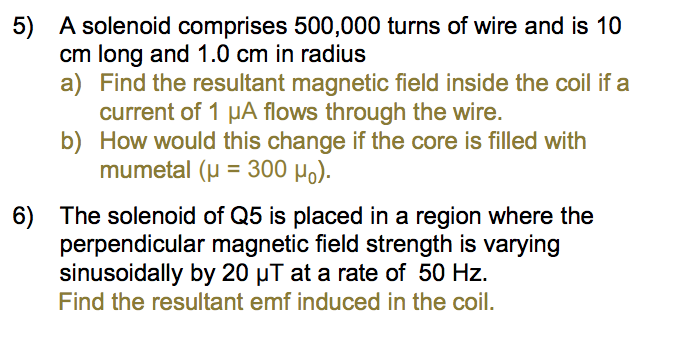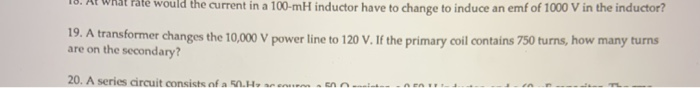Question

How many turns of wire would be required to make a 120- mH mH inductor out of a 32.0- cm cm -long air-filled solenoid with a diameter of 6.2 cm?Hello sir i want make 1.2 mh inductor with 14 awg inductor 800 rms watt
source: Electronics
Hello sir i want make 1.2 mh inductor with 14 awg inductor 800 rms watt
source: Electronics

#### Earn Coins

Coins can be redeemed for fabulous gifts.

Similar Homework Help Questions
• ### a) (7pts) How many turns of wire would be required to make a 130-mH inductance out...a) (7pts) How many turns of wire would be required to make a 130-mH inductance out of a 30.0-cm-long coil with a diameter of 4.2 cm? Assume that the coil is in a vacuum. b) (7pts) A 2.0 A current passes through this coil. What potential difference is induced across the coil if the current drops to zero in 4.0 us? Assume the current changes linearly. c) (3pts) (This part is related to part b) At which point a or...

• ### An air-filled cylindrical inductor with a inductance of 28 mH has 3300 turns. It is 2.8...

An air-filled cylindrical inductor with a inductance of 28 mH has 3300 turns. It is 2.8 cm in diameter. (a) What is its length? ____ cm (b) Assume a second cylindrical inductor with the same length and turns as the first, and a core filled with a material instead of air, but its diameter is 2.8 cm. If the second inductor has the same inductance as the first, what is the magnetic permeability of the material filling the second inductor...

• ### Suppose you wish to make a solenoid whose self-inductance is 1.2 mH. The inductor is to...

Suppose you wish to make a solenoid whose self-inductance is 1.2 mH. The inductor is to have a cross-sectional area of 2.00 10-3 m2 and a length of 0.053 m. How many turns of wire are needed?

• ### Suppose you wish to make a solenoid whose self-inductance is 1.4 mH. The inductor is to...

Suppose you wish to make a solenoid whose self-inductance is 1.4 mH. The inductor is to have a cross-sectional area of 1.7 x 10-3 m2 and a length of 0.059 m. How many turns of wire are needed?

• ### Titanium is a reasonably good conductor. A student wants to create an 8.00 mH inductor using...

Titanium is a reasonably good conductor. A student wants to create an 8.00 mH inductor using titanium wire. To do this, the student wraps the wire into the shape of a solenoid with radius 12.0 mm and length 1.50 cm. This means the student needs 460 turns of wire wrapped around this solenoid. The student uses very thin 28 AWG wire which has a diameter of 0.320 mm. The resulting inductor uses so much wire that it has a resistance...

• ### How many turns are required to make a solenoid of length .150m and cross sectional area...

How many turns are required to make a solenoid of length .150m and cross sectional area .00325m^2 with a self inducatance of 12.5 mH?

• ### A 62.5 m length of insulated copper wire is wound to form a solenoid of radius 2.3 cm. The copper wire has a radius of 0.51mm. (Assume the resistivity of copper is ρ = 1.7 ✕ 10−8 Ω · m.) (a) What is t...

A 62.5 m length of insulated copper wire is wound to form a solenoid of radius 2.3 cm. The copper wire has a radius of 0.51mm. (Assume the resistivity of copper is ρ = 1.7 ✕ 10−8 Ω · m.) (a) What is the resistance of the wire? Ω (b) Treating each turn of the solenoid as a circle, how many turns can be made with the wire? turns (c) How long is the resulting solenoid? m (d) What is...

• ### 5) A solenoid comprises 500,000 turns of wire and is 10 cm long and 1.0 cm...5) A solenoid comprises 500,000 turns of wire and is 10 cm long and 1.0 cm in radius. a) Find the resultant magnetic field inside the coil if a current of 1 μA flows through the wire. b) How would this change if the core is filled with mumetal (μ = 300 μ0 ). 6) The solenoid of Q5 is placed in a region where the perpendicular magnetic field strength is varying sinusoidally by 20 μT at a rate of...

• ### The inductance of a solenoid 10.0cm long with a cross-sectional area of 1,00 x 104 m2 is 0.400 mH. How many turns of wire does this solenoid have?

Question 6 The inductance of a solenoid 10.0cm long with a cross-sectional area of 1,00 x 104 m2 is 0.400 mH. How many turns of wire does this solenoid have? 318,000  159,000  564  282 Question 12 A 4 Ohms resistor is connected in parallel with a 20 resistor? 2.0A  2/3 A  3.0A  4/3 A A solenoid with a certain number of turns and carrying a current of 2A has a length of 34cm. If the magnitude of the magnetic field generated at the center of the...

• ### 18. Al wat rate would the current in a 100-mH inductor have to change to induce...18. Al wat rate would the current in a 100-mH inductor have to change to induce an emf of 1000 V in the inductor? 19. A transformer changes the 10,000 V power line to 120 V. If the primary coil contains 750 turns, how many turns are on the secondary? 20. A series circuit consists of a H an - -- - -- 19. A transformer changes the 10,000 V power line to 120 V. If the primary coil contains...